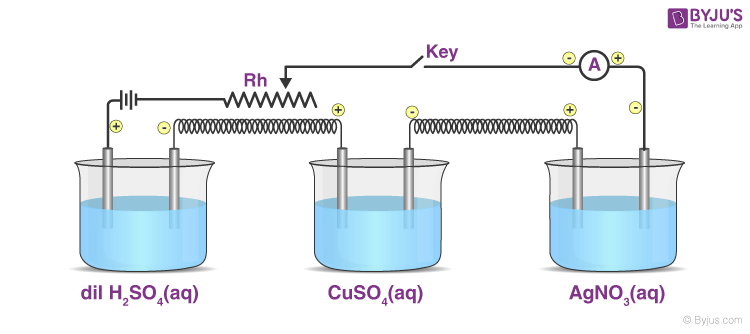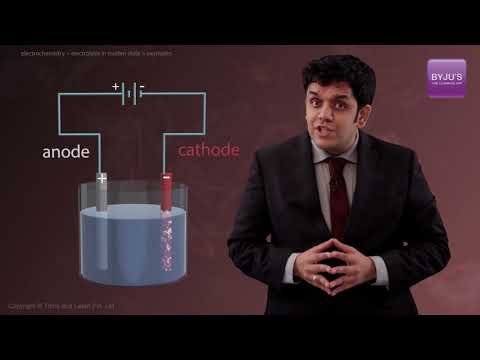## What is an Electrolytic Cell?

The electrochemical cell which facilitates a chemical reaction through the induction of electrical energy is known as an electrolytic cell.

This process of carrying out non-spontaneous reactions under the influence of electric energy is termed as electrolysis.Michael Faraday conducted an extensive investigation on electrolysis of solutions and melts of electrolytes. He was the first scientist who described the quantitative aspects of the Laws of Electrolysis. He proposed two laws to explain the quantitative aspects of electrolysis popularly known as Faraday’s laws of electrolysis namely first law of electrolysis and the second law of electrolysis.

## Recommended Videos

#### Electrochemistry – Electrolytic Cell and Electrolysis#### Galvanic Cell, Electrolytic Cell, EMF of Cell & Faraday’s Laws## Faraday’s – First Law of Electrolysis

It is one of the primary laws of electrolysis. It states, during electrolysis, the amount of chemical reaction which occurs at any electrode under the influence of electrical energy is proportional to the quantity of electricity passed through the electrolyte.

## Faraday’s – Second Law of Electrolysis

Faraday’s second law of electrolysis states that if the same amount of electricity is passed through different electrolytes, the masses of ions deposited at the electrodes are directly proportional to their chemical equivalents.

From these laws of electrolysis, we can deduce that the amount of electricity needed for oxidation-reduction depends on the stoichiometry of the electrode reaction.

For example,

$$\begin{array}{l}Na^{+} + e^{-}~\rightarrow~ Na\end{array}$$

As we can observe, one mole of the electron is required for the reduction of one mole of sodium ions. We know that charge on one electron is equal to

$$\begin{array}{l}1.6021~× ~10^{–19}C\end{array}$$

Therefore, the charge on one mole of electrons is equal to:

$$\begin{array}{l}N_A~×~ 1.6021~ ×~ 10^{–19} C = 6.02~ ×~ 10^{23} mol^{–1}~× ~1.6021 ~× ~10^{–19}C = 96487~ C~ mol^{–1}\end{array}$$

This quantity of electricity is defined as one Faraday and is denoted by F. Hence; one Faraday is defined as the charge carried per unit mole of electrons.

The product of an electrolytic reaction depends on the nature of the material being electrolysed and the type of electrodes used. In the case of an inert electrode such as platinum or gold, the electrode does not participate in the chemical reaction and acts only as a source or sink for electrons. While, in the case of a reactive electrode, the electrode participates in the reaction.

Hence, different products are obtained for electrolysis in the case of reactive and inert electrodes. Oxidizing and reducing species present in the electrolytic cell and their standard electrode potential too, affect the products of electrolysis.

## Related Videos

#### Electrolysis in Molten State#### Faraday’s Law of Electromagnetic Induction## Frequently Asked Questions – FAQs

Q1

The Faraday is an electric charge volume unit without measurements, equal to approximately 6.02 x 1023 electric charge carriers.
Q2

### Why is Faraday’s law important?

A shifting magnetic flux creates an electric field, according to Faraday’s law. Faraday’s law is particularly important since it addresses the connection of the E-field and the B-field and understands that this connection necessitates flux fluctuation over time.
Q3

### How does electrolysis remove rust?

Electrolysis is a method of removing iron oxide by passing a small electrical charge through the rusty metal from a battery or battery charger to induce ion exchange while the device is submerged in an electrolyte solution.
Q4

### What happens to water during electrolysis?

Water’s Electrolysis. By passing an electrical current through it, water can be decomposed. When this happens, an oxidation-reduction reaction is caused by the electrons from the electric current.
Q5

### What is the negative electrode called in electrolysis?

Through electrolysis, the negatively charged electrode is called the cathode. The positively charged electrode is called the anode in electrolysis. Negatively charged ions are moving towards the anode.

For a detailed discussion on electrolysis and the Faraday’s laws of electrolysis, download BYJU’S – The Learning App.’

Test your knowledge on Laws Of Electrolysis